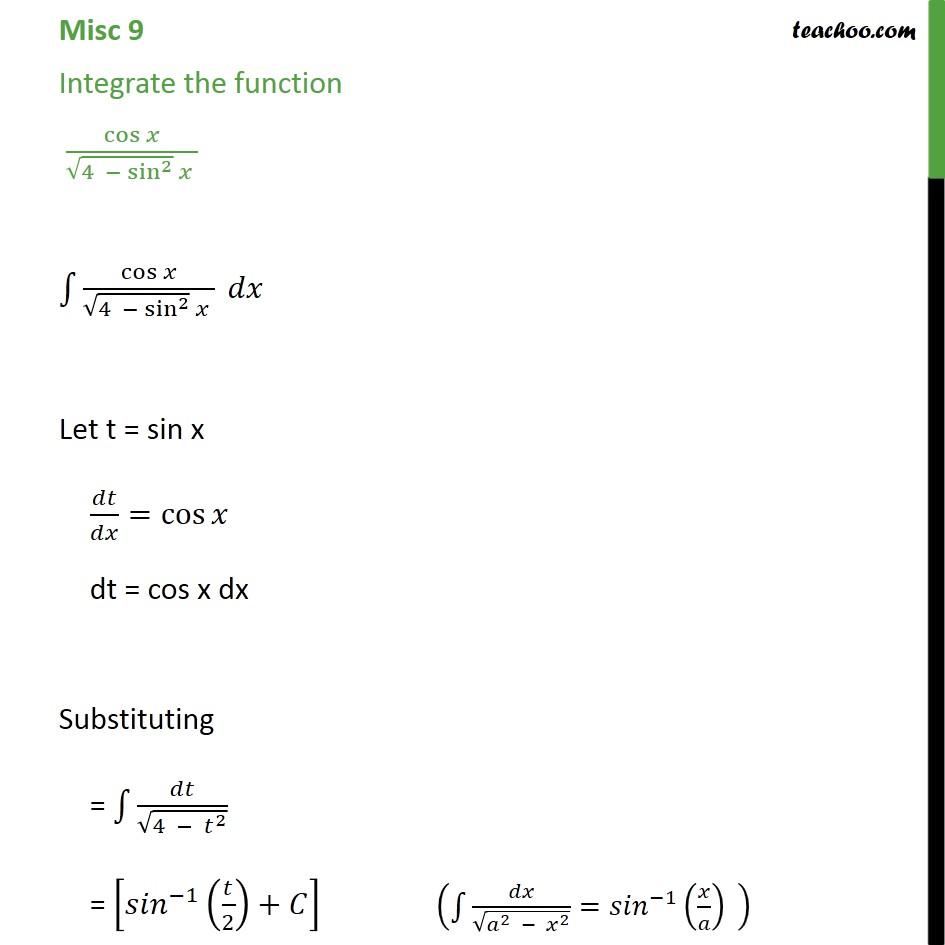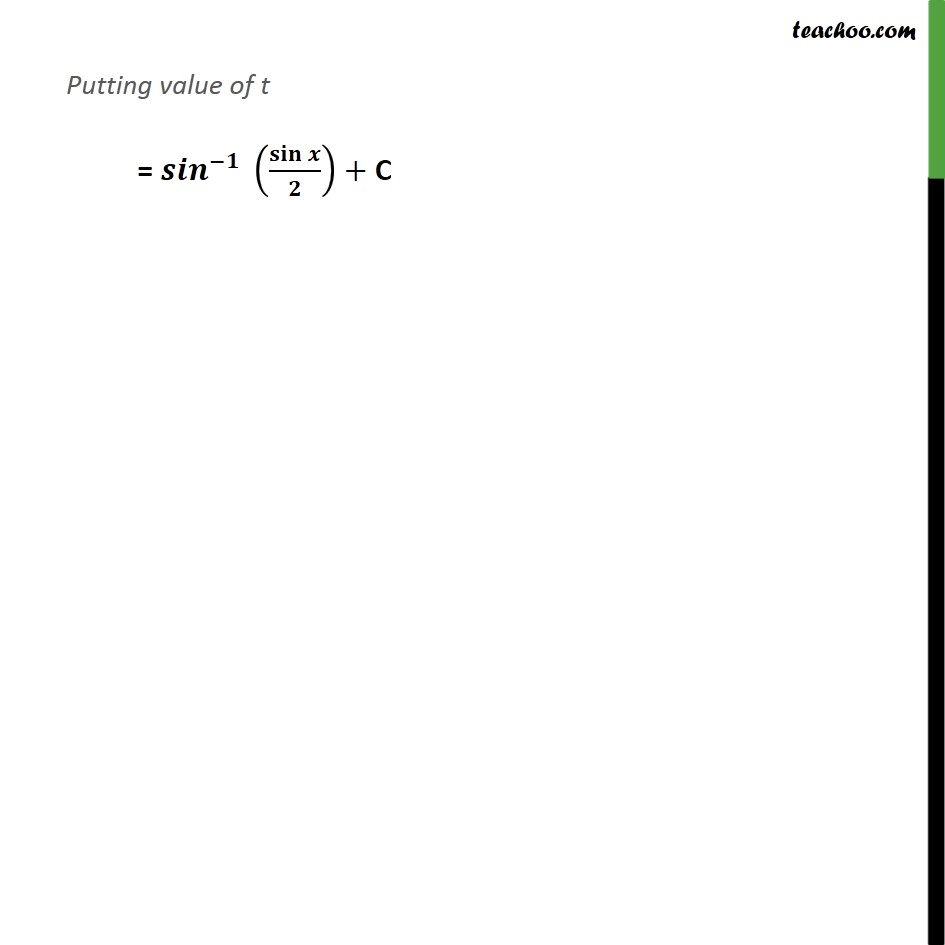1. Chapter 7 Class 12 Integrals (Term 2)
2. Serial order wise
3. Miscellaneous

Transcript

Misc 9 Integrate the function cos 4 sin 2 cos 4 sin 2 Let t = sin x = cos dt = cos x dx Substituting = 4 2 = 1 2 + Putting value of t = + C